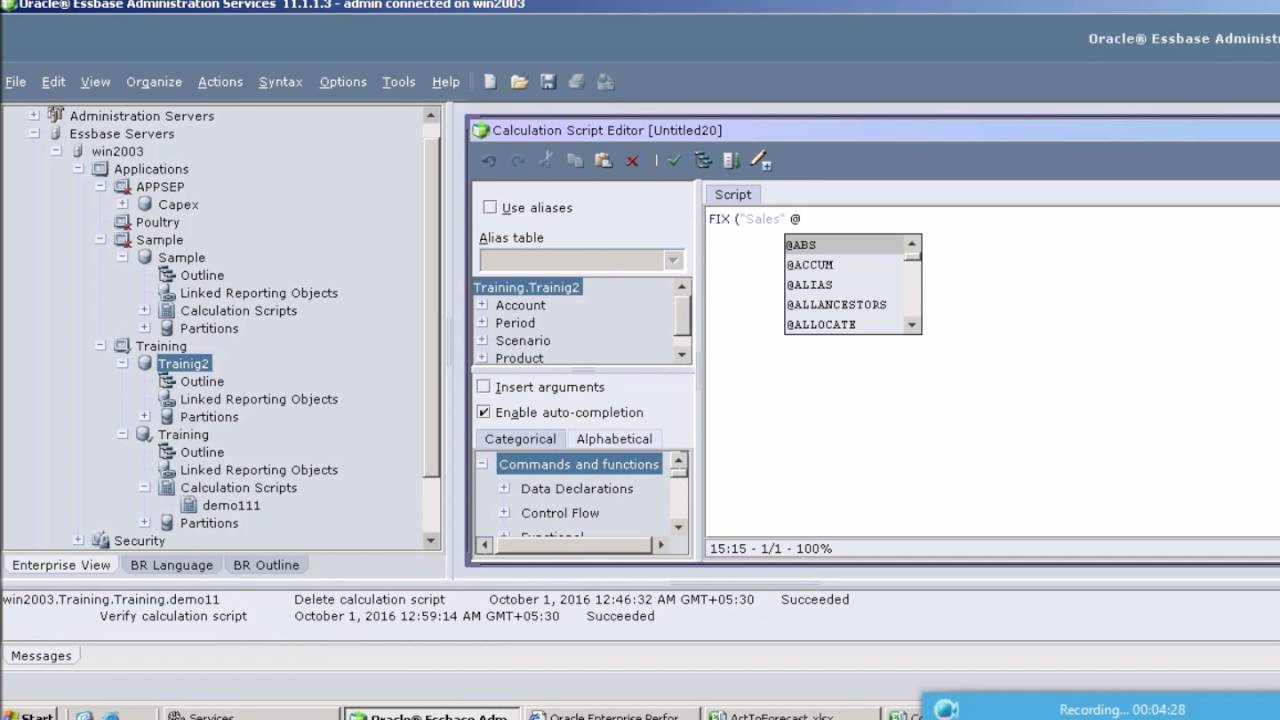# Blog

## Essbase Technical Reference Series: Calculation Operators

• , Business AnalystI'm sure most readers of this blog are quite aware of just how powerful Oracle's Essbase really is, but with so many capabilities, many functions and commands are often lost on even the most experienced Essbase administrators or analysts. Well, it's time to begin by reviewing your calculation operators! Calculation operators are the backbone of working with Essbase formulas, and knowing what they are capable of will only improve your understanding of the system and possible solutions to implement.

Just a short review, Essbase Calculation Operators, being Mathematical, Conditional and Logical, and Cross-Dimensional, define equations for Essbase member formulas and calculation scripts. There really aren't that many to remember but their uses are nearly endless.

### Starting off with the Mathematical Operators, the basics:

• + : guess what? It adds things...
• - : surprise! It subtracts.
• * : of course multiplies .
• / : intuitively divides.
• % : getting a little more interesting, evaluates percentages (member1%member2 evaluates member1 as a percentage of member2).
• () : Lastly, controls the order of calculations and nests equations and formulas.

#### Moving on to the fun stuff now, Conditional and Logical Operators:

IF | ELSE | ELSEIF |ENDIF : They test conditions and calculate a formula based on the success or failure of the test.

• (>) : Data value is greater than.
• (>=) : Data value is greater than or equal to.
• (<) : Data value is less than.
• (<=) : Data value is less than or equal to.
• (= =) : Data value is equal to (note the two equals!).
• (< >) or != : Data value is not equal to.

AND : Logical AND linking operator for multiple value tests. Results if TRUE if both conditions are TRUE. Otherwise, the result is FALSE.*

OR : Logical OR linking operator for multiple value tests. Result is TRUE if either condition is TRUE. Otherwise the result is FALSE.*

NOT : Logical NOT operator. Result is TRUE if condition is FALSE. Result is FALSE if condition is TRUE.*

*Remember! The logical constants TRUE and FALSE are interpreted as 1 (TRUE) and 0 (FALSE) where appropriate.

Ok... so right now most of you are wondering why I'm explaining basic mathematical signage and English to you, but hey, everyone has to start from point 0!

Lastly, the fairly simple Cross-Dimensional Operators:

Every time you see or write a ( -> ), know that it points to data values of specific member combinations. Basically, if you have a ( - ) and a ( > ) together without a space in between, it's indicating the connection between dimensions for an intersection.

Here's an example:

ELSEIF(@ISUDA("Entity","AUD_Rev"))

Now, this is a common example where a currency exchange rate is stored at a particular intersection, and that intersection is referenced for a currency conversion calculation.

That's all on the basics for now folks, be sure to stay tuned as we get into a bit more detail!

#### Contact MindStream Analytics

Want to know more about Essbase? Please complete the form below and we'll get back to you shortly.

#### Partner SpotLightOracle

Oracle has the most comprehensive suite of integrated, global business applications that enable organizations to make better decisions, reduce cost